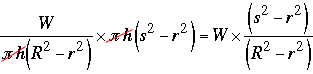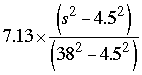SEARCH HOMEMath Central Quandaries & QueriesQuestion from james: I am after a standard calculation so that after each usage of the Reel I can get the Remaining Quantity in kilos left on the roll below is an example of roll THICKNESS: 30 MICRON REEL WIDTH: 7.5 CM REEL DIAMETER: 76 CM REEL WEIGHT: 7.13 KILOS The core centre has a 9cm diameter, the weight of the roll is excluding the core , and after each use the diameter of the role is measured. I am a factory supervisor working on project and need this calculation info for template.. Thanks JamesJames,

The volume of a cylinder is given by π r2 h cubic units where r is the radius, h is the height and π = 3.1416. Suppose R is the radius of the roll, r is the radius of the core and h is the height of the roll. For your example R = 76/2 = 38, r = 9/2 = 4.5 and h = 7.5, all in centimeters. The volume of film on the roll is then

original volume = π R2h - π r2 h = π h (R2 - r2) cubic units.

Suppose the mass is W units (for your example W = 7.13 kilos). The density of the film is then

W/(original volume) mass units/(cubic length units).

7.13/[π × 7.5 (382 - 4.52)] kilos per cubic centimeter.

Suppose that, after a use, the radius of the film remaining on the roll is s units. The volume of film remaining on the roll is then

new volume = π s2h - π r2 h = π h (s2 - r2) cubic units.

Since the density is [W/(original volume)] mass units/(cubic length units) the mass of the reduced roll will be

[W/(original volume)] × (new volume) =mass units.

For your example that would bekilos.

HarleyMath Central is supported by the University of Regina and The Pacific Institute for the Mathematical Sciences.